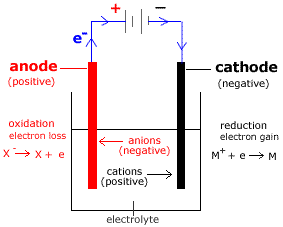Electrolysis of Molten, or Liquid, Salts Chemistry Tutorial

Key Concepts

• Melting a salt enables it to conduct electricity.

non-conductor conductor
MX(s) heat
M+(l) + X-(l)

• The use of inert electrodes, electrodes made of a material that will not take part in the reactions, means the only species present that can take part in the electrolytic cell reactions are the anions and cations of which the salt is composed.
• During the electrolysis of a molten salt:
(a) Oxidation occurs at the anode (positive electrode).
(Oxidation, loss of electrons, occurs at the anode)

(b) Anions (negatively charged ions) migrate to the positive anode (anode).

(c) Reduction occurs at the cathode (negative electrode).
(Reduction, gain of electrons, occurs at the cathode)

(d) Cations (positively charged ions) migrate to the negative cathode (cathode).

• Reactions occurring during electrolysis of a molten, or liquid, salt (MX(l)) are:

 oxidation at anode (positive electrode) reduction at cathode (negative electrode) X-(l) → X + e- e- + M+(l) → M

• Electrolysis is a non-spontaneous reaction:

Ecell for the electrolytic cell is negative1.

• Applied emf must be greater than the emf for the cell, ie greater than -Ecell.
• Mass of substance produced electrolytically is proportional to the quantity of electricity flowing.

No ads = no money for us = no free stuff for you!

Electrolytic Cell for the Electrolysis of a Molten Salt

For the electrolysis of molten salt, MX(l) using inert electrodes:

• The electrolyte is MX(l).
• M+ are the cations which will be reduced at the cathode.
• X- are the anions which will be oxidized at the anode.NOTE: Electrons (e-) flow from anode (positive electrode) to cathode (negative electrode) Anions (negative ions) flow to the anode. Cations (positive ions) flow to the cathode.

Electrolytic Cell Reactions
anode:
(oxidation)
X- X + e-   E1
cathode:
(reduction)
M+ + e- M   E2

cell:
(REDOX)
X- + M+ X + M   Ecell = E1 + E2
Ecell = -V
Ecell is negative

EMF (volts) required to drive this non-spontaneous reaction is greater than Ecell for the spontaneous redox reaction.
Required voltage (EMF) > -E(electrolytic cell)

Do you know this?

Play the game now!

Worked Example : Electrolysis of NaCl(l)

Sodium chloride, NaCl, is a salt composed of sodium ions, Na+, and chloride ions, Cl-.

Sodium chloride, NaCl, can not conduct electricity when it is present as a solid, NaCl(s), because the ions are locked into a regular three dimensional lattice are are not free to move.

When heat is applied to the solid sodium chloride, NaCl(s), it will melt to form liquid sodium chloride, NaCl(l) :

solid heat
liquid
NaCl(s) heat
NaCl(l)

In the liquid state, the sodium ions (Na+(l)) and chloride ions (Cl-(l)) are free to move (they are said to be be mobile), so molten, or liquid, sodium chloride can conduct electricity.

This means it is possible to construct an electrolytic cell using molten sodium chloride as shown in the diagram below:

 Na+ → ← Cl-

Note:

• The electrodes must be inert electrodes so that there are no competing reactions in the electrolytic cell.
• Electrons flow from anode (positive electrode) to cathode (negative electrode).
• Anions, Cl-, flow towards the anode.
• Cations, Na+, flow towards the cathode.

Remember, oxidation occurs at the anode and reduction occurs at the anode, so we can write the half-equations for the reactions at the anode and cathode, and therefore the overall redox equation for the redox reaction occurring in this electrolytic cell:

Electrolytic Cell Reactions
anode:
(oxidation)
Cl- ½Cl2(g) + e-
cathode:
(reduction)
Na+ + e- Na

cell:
(REDOX)
Cl- + Na+ ½Cl2(g) + Na

Bubbles of Cl2(g) would be produced at the anode.
Droplets of Na(l) would be produced at the cathode.

Do you understand this?

Take the test now!

1. The species are NOT present in their standard states so that the electrode potentials (E) are NOT standard electrode potentials (Eo).